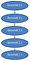# Factorial Program Algorithm Analysis

The Factorial Algorithm is great for recursion

# What is factorial ?

In mathematics, the factorial of a non-negative integer n, denoted by n!, is the product of all positive integers less than or equal to n. For example
5! = 5 x 4 x 3 x 2 x 1 = 120. It is just the product of an integer and all the integers below it.-Wikepedia

# How to write a factorial function using recursion ?

The factorial program can be written recursively, because it is a function that calls itself.

Pseudo Code
1. func factorial(n)
2. if (n == 1)
3. return 1
4. return n *
factorial(n -1)

C-Program

`factorial(int n){	//Base Case	if(n == 1)	return 1;		return n *factorial(n-1);}`

You can see how to convert a function to recurrence relation in this video, it’s not the factorial function, but a function with the same recurrence relation.

# Write the factorial function as a recurrence relation

This function written as a recurrence relation is:

T(n)=1 for n=0
T(n)=1+T(n-1) for n>0

This recurrence running time in Big O notation is: **O(n)**, which is the main purpose for converting algorithms to recurrence relations.
you can learn how to solve this from this video

Let’s look at an example:
Suppose we had a arbitrary number n=5, so we use the function factorial(5)

We get the following Number of iterations
1) factorial(5) = 5 * factorial(4)
2) factorial(4) = 4 * factorial(3)
3) factorial(3) = 3 * factorial(2)
4) factorial(2) = 2 * factorial(1)
5) factorial(1) = 1The recursion performed by the factorial function 5! takes only 5 steps. This means the factorial function is O(n) since our input size “n”= 5 and it took only 5 steps

So the factorial function called itself 5 times which is the exact same number of the input size’n’, where n = 5. So the recurrence ran ’n’ times, and is therefore O(n).

Thanks for reading this article I hope it’s helpful to you all ! Keep up the learning, and if you would like more algorithm analysis videos please visit and subscribe to my YouTube channels (randerson112358 & compsci112358 )

# Check Out the following for more content / videos on Algorithm Analysis and Programming:

compsci112358:

Video Tutorials on Recurrence Relation:

Video Tutorial on Algorithm Analysis:
https://www.udemy.com/algorithm-analysis/

Written by